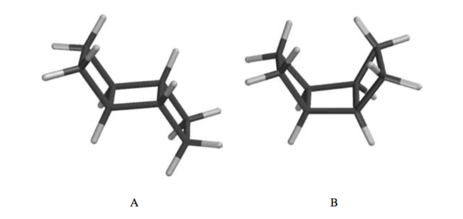# Problem: One of the following two stereoisomers is 20 kJ/mol (4.9 kcal/mol) less stable than the other. Indicate which isomer is the less stable, and identify the reason for its decreased stability.

###### Problem Details

One of the following two stereoisomers is 20 kJ/mol (4.9 kcal/mol) less stable than the other. Indicate which isomer is the less stable, and identify the reason for its decreased stability.What scientific concept do you need to know in order to solve this problem?

Our tutors have indicated that to solve this problem you will need to apply the Ring Strain concept. You can view video lessons to learn Ring Strain. Or if you need more Ring Strain practice, you can also practice Ring Strain practice problems.

What is the difficulty of this problem?

Our tutors rated the difficulty ofOne of the following two stereoisomers is 20 kJ/mol (4.9 kca...as low difficulty.

How long does this problem take to solve?

Our expert Organic tutor, Jonathan took undefined 40 seconds to solve this problem. You can follow their steps in the video explanation above.

What professor is this problem relevant for?

Based on our data, we think this problem is relevant for Professor Daoudi's class at UCF.

What textbook is this problem found in?

Our data indicates that this problem or a close variation was asked in Organic Chemistry - Carey 8th Edition. You can also practice Organic Chemistry - Carey 8th Edition practice problems.